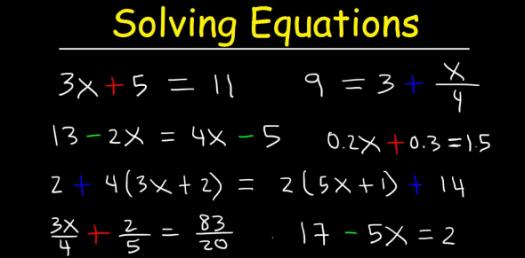# Can You Solve These Basic Equations? Math Trivia Quiz

23 Questions | Total Attempts: 27SettingsCan you solve these basic equations? Not everyone has a smooth time when it comes to solving some common math problems, and it is for this reason that there are so many math quizzes around designed to keep out minds fresh, and the one below is one of them. How about you check it out and get to polish up your skills.

• 1.
What is the inverse operation for multiplication?
• A.

• B.

Subtraction

• C.

Division

• 2.
What is the inverse operation for addition?
• A.

Subtraction

• B.

Multiplication

• C.

Division

• 3.
-15 = x / 4
• A.

-60

• B.

60

• C.

-11

• D.

-19

• 4.
21 + m = 4
• A.

25

• B.

17

• C.

-17

• D.

84

• 5.
6n = 30
• A.

5

• B.

-5

• C.

24

• D.

180

• 6.
9 = x / 18
• A.

-162

• B.

2

• C.

162

• D.

11

• 7.
-5 = -20 + x
• A.

-15

• B.

-25

• C.

100

• D.

15

• 8.
11x = -286
• A.

297

• B.

26

• C.

-297

• D.

-26

• 9.
-5 = x / 12
• A.

50

• B.

-50

• C.

-60

• D.

-17

• 10.
X + 8 = 15
• A.

X = 7

• B.

X = -7

• C.

X = 23

• D.

X = -23

• 11.
Y - 3 = -5
• A.

Y = 8

• B.

Y = -8

• C.

Y = 2

• D.

Y = -2

• 12.
B + 24 = 19
• A.

B = 5

• B.

B = -43

• C.

B = 43

• D.

B = -5

• 13.
3x = 12
• A.

X = -4

• B.

X = 4

• C.

X = 36

• D.

X = 15

• 14.
-2x = 88
• A.

X = 44

• B.

X = -44

• C.

X = -176

• D.

X = 176

• 15.
M + 43 = 100
• A.

M = 57

• B.

M = 143

• C.

M = -143

• D.

M = -57

• 16.
H - 34 = 17
• A.

H = 17

• B.

H = 51

• C.

H = -17

• D.

H = -51

• 17.
7d = 56
• A.

D = -8

• B.

D = 7

• C.

D = 8

• D.

D = 56

• 18.
R / 5 = -9
• A.

R = 5

• B.

R = 9

• C.

R = -45

• D.

R = 45

• 19.
Y / 12 = -11
• A.

Y = -132

• B.

Y = 12

• C.

Y = 1

• D.

Y = 132

• 20.
-3m = -66
• A.

198

• B.

-22

• C.

22

• D.

69

• 21.
9 + x = 2
• A.

7

• B.

-11

• C.

-7

• D.

11

• 22.
-12 = x / 3
• A.

4

• B.

-36

• C.

-4

• D.

36

• 23.
-3 + x = -12
• A.

-15

• B.

15

• C.

-9

• D.

9

Related TopicsBack to top